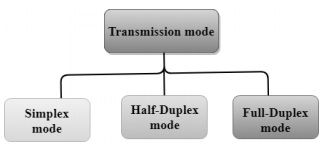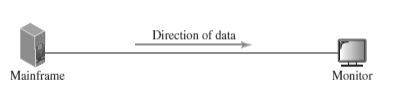# What is simplex mode of transmission in computer networks?

When a data is transmitted from one device to another device is known as transmission mode, it is also known as the communication mode.

Generally, the communication channel is a direction associated, and transmission media role is to provide the direction. So, we call transmission mode a directional mode.

The transmission mode is work in the physical layer.

## Types of Transmission mode

The Transmission mode is divided into three categories, which are as follows −

• Simplex Mode
• Half-duplex Mode
• Full-duplex mode (Duplex Mode)

The types of transmission mode are shown in the chart below −Let us see the simplex mode of transmission.

## Simplex mode

In Simplex mode, the communication is unidirectional, that means the data will flow in one direction.

A device can send the data but cannot receive it or it can receive the data but cannot send the data.

This type of transmission mode is not very popular because the communications require the two-way exchange of data.

## Examples

The simplex mode is used in the business field as in sales that do not require any corresponding reply.

The radio station is a simplex channel as it transmits the signal to the listeners but never allows them to transmit back.

Keyboard and Monitor are the examples of the simplex mode as a keyboard can only accept the data from the user and monitor can only be used to display the data on the screen

Given below is the diagram of simplex mode −The advantages of simplex are as follows −

• The main advantage is that the full capacity of the communication channel can be utilised during transmission.

• In simplex mode, the station can utilize the entire bandwidth of the communication channel, so that more data can be transmitted at a time.

The only disadvantage of simplex is that the communication is unidirectional. So it has no inter-communication between devices.

## Concept

Let us work on small concept as given below −

The Communication system of simplex mode has data transmissions rate as 16 Mbps. Let the content of transmission be ASCII text. Calculate the number of characters the system transmits in 2 seconds.

## Solution

Size of one ASCII char = 1byte = 8 bits

Data transmissions rate = 16 Mbps

Therefore

Total data transmission in 2 seconds = 16*2 = 32Mb

Since, 1M = 106

Total number of transmit = (32*106) / 8

=> 4 * 106 = 4000000 characters